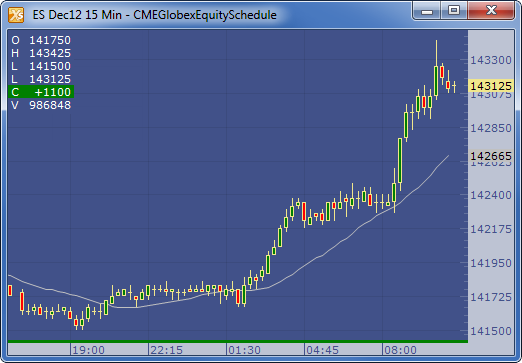← Back to X_TRADER® Help Library

### X_STUDY® Documentation

#### Simple Moving Average (SMA)

##### Description

The Simple Moving Average (SMA) is calculated by adding the price of an instrument over a number of time periods and then dividing the sum by the number of time periods. The SMA is basically the average price of the given time period, with equal weighting given to the price of each period.

##### Formula

SMA = ( Sum ( Price, n ) ) / n

Where: n = Time Period

##### Example